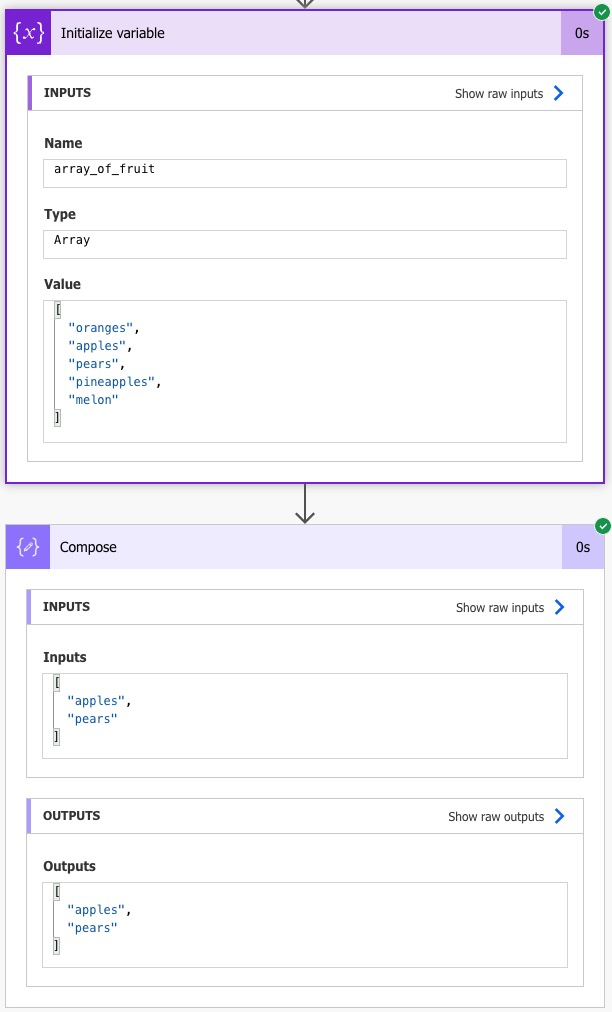## Power Automate Array Slicing

Recently, I built a power automate flow where I needed a subset of an array. Here are the key takeaways from my experience.

Array slicing is the process of extracting a specific subset of an existing array. Let's say we have the following array of strings:

``````array_of_fruit = ["oranges", "apples", "pears", "pineapples", "melon"]
``````

If we want to extract only "apples" and "pears" from the array, then the resulting slice of the array would be:

``````favorite_fruits = ["apples", "oranges"]
``````

In Python, we can easily achieve this by using indexing:

``````favorite_fruits = array_of_fruit[1:2]
``````

However, in Power Automate, we need to utilize Power FX code like so:

``````take(skip([array],start_count),amount_of_items)
``````

In the above snippet, `start_count` is the index you want your subset to start, and `amount_of_items` is the number of items you want to extract from your array.

For example, if we want to extract the same subset of the array as our Python example above, we can use the following Power FX code:

``````take(skip(variable('array_of_strings'),1),2)
``````

Would return the same array as the Python variable `favorite_fruits`.

Screenshot:Posted on 2023-04-04